Normal view

# Loss models : from data to decisions / Stuart A. Klugman, Harry H. Panjer, Gordon E. Willmot.

Material type:TextLanguage: English Publication details: c2012. Edition: 4th edDescription: xiv, 512 p. : ill. ; 26 cmISBN: 9781118315323; 1118315324DDC classification: 368.012 LOC classification: HG8781 | .K583 2012Online resources: WorldCat details | E-book Fulltext
Contents:
TOC Preface xiii PART I INTRODUCTION 1 Modeling 3 1.1 The model-based approach 3 1.2 Organization of this book 5 2 Random variables 7 2.1 Introduction 7 2.2 Key functions and four models 9 3 Basic distributional quantities 19 3.1 Moments 19 3.2 Percentiles 27 3.3 Generating functions and sums of random variables 29 3.4 Tails of distributions 31 3.5 Measures of Risk 38 PART II ACTUARIAL MODELS 4 Characteristics of Actuarial Models 49 4.1 Introduction 49 4.2 The role of parameters 49 5 Continuous models 59 5.1 Introduction 59 5.2 Creating new distributions 59 5.3 Selected distributions and their relationships 72 5.4 The linear exponential family 75 6 Discrete distributions 79 6.1 Introduction 79 6.2 The Poisson distribution 80 6.3 The negative binomial distribution 83 6.4 The binomial distribution 85 6.5 The (a, b, 0) class 86 6.6 Truncation and modification at zero 89 7 Advanced discrete distributions 95 7.1 Compound frequency distributions 95 7.2 Further properties of the compound Poisson class 101 7.3 Mixed frequency distributions 107 7.4 Effect of exposure on frequency 114 7.5 An inventory of discrete distributions 114 8 Frequency and severity with coverage modifications 117 8.1 Introduction 117 8.2 Deductibles 117 8.3 The loss elimination ratio and the effect of inflation for ordinary deductibles 122 8.4 Policy limits 125 8.5 Coinsurance, deductibles, and limits 127 8.6 The impact of deductibles on claim frequency 131 9 Aggregate loss models 137 9.1 Introduction 137 9.2 Model choices 140 9.3 The compound model for aggregate claims 141 9.4 Analytic results 155 9.5 Computing the aggregate claims distribution 159 9.6 The recursive method 161 9.7 The impact of individual policy modifications on aggregate payments 173 9.8 The individual risk model 176 PART III CONSTRUCTION OF EMPIRICAL MODELS 10 Review of mathematical statistics 187 10.1 Introduction 187 10.2 Point estimation 188 10.3 Interval estimation 196 10.4 Tests of hypotheses 198 11 Estimation for complete data 203 11.1 Introduction 203 11.2 The empirical distribution for complete, individual data 207 11.3 Empirical distributions for grouped data 211 12 Estimation for modified data 217 12.1 Point estimation 217 12.2 Means, variances, and interval estimation 225 12.3 Kernel density models 236 12.4 Approximations for large data sets 240 PART IV PARAMETRIC STATISTICAL METHODS 13 Frequentist estimation 253 13.1 Method of moments and percentile matching 253 13.2 Maximum likelihood estimation 259 13.3 Variance and interval estimation 272 13.4 Non-normal confidence intervals 280 13.5 Maximum likelihood estimation of decrement probabilities 282 14 Frequentist Estimation for discrete distributions 285 14.1 Poisson 285 14.2 Negative binomial 289 14.3 Binomial 291 14.4 The (a, b, 1) class 293 14.5 Compound models 297 14.6 Effect of exposure on maximum likelihood estimation 299 14.7 Exercises 300 15 Bayesian estimation 305 15.1 Definitions and Bayes theorem 305 15.2 Inference and prediction 309 15.3 Conjugate prior distributions and the linear exponential family 320 15.4 Computational issues 322 16 Model selection 323 16.1 Introduction 323 16.2 Representations of the data and model 324 16.3 Graphical comparison of the density and distribution functions 325 16.4 Hypothesis tests 330 16.5 Selecting a model 342 PART V CREDIBILITY 17 Introduction and Limited Fluctuation Credibility 357 17.1 Introduction 357 17.2 Limited fluctuation credibility theory 359 17.3 Full credibility 360 17.4 Partial credibility 363 17.5 Problems with the approach 366 17.6 Notes and References 367 17.7 Exercises 367 18 Greatest accuracy credibility 371 18.1 Introduction 371 18.2 Conditional distributions and expectation 373 18.3 The Bayesian methodology 377 18.4 The credibility premium 385 18.5 The Buhlmann model 388 18.6 The Buhlmann-Straub model 392 18.7 Exact credibility 397 18.8 Notes and References 401 18.9 Exercises 402 19 Empirical Bayes parameter estimation 415 19.1 Introduction 415 19.2 Nonparametric estimation 418 19.3 Semiparametric estimation 428 19.4 Notes and References 430 19.5 Exercises 430 PART VI SIMULATION 20 Simulation 437 20.1 Basics of simulation 437 20.2 Simulation for specific distributions 442 20.3 Determining the sample size 448 20.4 Examples of simulation in actuarial modeling 450 Appendix A: An inventory of continuous distributions 459 A.1 Introduction 459 A.2 Transformed beta family 463 A.3 Transformed gamma family 467 A.4 Distributions for large losses 470 A.5 Other distributions 471 A.6 Distributions with finite support 473 Appendix B: An inventory of discrete distributions 475 B.1 Introduction 475 B.2 The (a, b, 0) class 476 B.3 The (a, b, 1) class 477 B.4 The compound class 480 B.5 A hierarchy of discrete distributions 482 Appendix C: Frequency and severity relationships 483 Appendix D: The recursive formula 485 Appendix E: Discretization of the severity distribution 487 E.1 The method of rounding 487 E.2 Mean preserving 488 E.3 Undiscretization of a discretized distribution 488 Appendix F: Numerical optimization and solution of systems of equations 491 F.1 Maximization using Solver 491 F.2 The simplex method 495 F.3 Using Excel(R) to solve equations 496 References 501
Summary: Thoroughly revised and updated with essential material related to the C/4 actuarial exam, this invaluable new edition maintains an approach to modeling and forecasting utilizing tools related to risk theory, loss distributions, and survival models.
Tags from this library: No tags from this library for this title.
Star ratingsAverage rating: 0.0 (0 votes)
Holdings
Item type Current library Collection Call number Copy number Status Date due Barcode Item holdsE-Book
E-book
Non-fiction 368.012 KLL 2012 (Browse shelf(Opens below)) Not for loanText
Reserve Section
Non-fiction 368.012 KLL 2012 (Browse shelf(Opens below)) C-1 Not For Loan 27378Text
Circulation Section
Non-fiction 368.012 KLL 2012 (Browse shelf(Opens below)) C-2 Available 28153
Total holds: 0
##### Browsing EWU Library shelves, Shelving location: Circulation Section Close shelf browser (Hides shelf browser)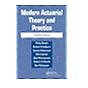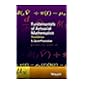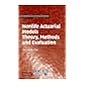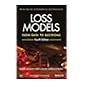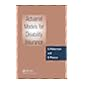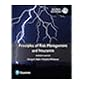No cover image available 368.01 MOD 2005 Modern actuarial theory and practice / 368.01 PEF 2015 Fundamentals of actuarial mathematics / 368.01 TSN 2009 Nonlife actuarial models : 368.012 KLL 2012 Loss models : 368.38601 HAA 1999 Actuarial models for disability insurance / 368 REP 2017 Principles of risk management and insurance / 370.19 JEE 1965 Educational sociology : an approach to its development as a practical field of study /

"Society of Actuaries."

Includes bibliographical references (p. 501-506) and index.

TOC Preface xiii PART I INTRODUCTION 1 Modeling 3 1.1 The model-based approach 3 1.2 Organization of this book 5 2 Random variables 7 2.1 Introduction 7 2.2 Key functions and four models 9 3 Basic distributional quantities 19 3.1 Moments 19 3.2 Percentiles 27 3.3 Generating functions and sums of random variables 29 3.4 Tails of distributions 31 3.5 Measures of Risk 38 PART II ACTUARIAL MODELS 4 Characteristics of Actuarial Models 49 4.1 Introduction 49 4.2 The role of parameters 49 5 Continuous models 59 5.1 Introduction 59 5.2 Creating new distributions 59 5.3 Selected distributions and their relationships 72 5.4 The linear exponential family 75 6 Discrete distributions 79 6.1 Introduction 79 6.2 The Poisson distribution 80 6.3 The negative binomial distribution 83 6.4 The binomial distribution 85 6.5 The (a, b, 0) class 86 6.6 Truncation and modification at zero 89 7 Advanced discrete distributions 95 7.1 Compound frequency distributions 95 7.2 Further properties of the compound Poisson class 101 7.3 Mixed frequency distributions 107 7.4 Effect of exposure on frequency 114 7.5 An inventory of discrete distributions 114 8 Frequency and severity with coverage modifications 117 8.1 Introduction 117 8.2 Deductibles 117 8.3 The loss elimination ratio and the effect of inflation for ordinary deductibles 122 8.4 Policy limits 125 8.5 Coinsurance, deductibles, and limits 127 8.6 The impact of deductibles on claim frequency 131 9 Aggregate loss models 137 9.1 Introduction 137 9.2 Model choices 140 9.3 The compound model for aggregate claims 141 9.4 Analytic results 155 9.5 Computing the aggregate claims distribution 159 9.6 The recursive method 161 9.7 The impact of individual policy modifications on aggregate payments 173 9.8 The individual risk model 176 PART III CONSTRUCTION OF EMPIRICAL MODELS 10 Review of mathematical statistics 187 10.1 Introduction 187 10.2 Point estimation 188 10.3 Interval estimation 196 10.4 Tests of hypotheses 198 11 Estimation for complete data 203 11.1 Introduction 203 11.2 The empirical distribution for complete, individual data 207 11.3 Empirical distributions for grouped data 211 12 Estimation for modified data 217 12.1 Point estimation 217 12.2 Means, variances, and interval estimation 225 12.3 Kernel density models 236 12.4 Approximations for large data sets 240 PART IV PARAMETRIC STATISTICAL METHODS 13 Frequentist estimation 253 13.1 Method of moments and percentile matching 253 13.2 Maximum likelihood estimation 259 13.3 Variance and interval estimation 272 13.4 Non-normal confidence intervals 280 13.5 Maximum likelihood estimation of decrement probabilities 282 14 Frequentist Estimation for discrete distributions 285 14.1 Poisson 285 14.2 Negative binomial 289 14.3 Binomial 291 14.4 The (a, b, 1) class 293 14.5 Compound models 297 14.6 Effect of exposure on maximum likelihood estimation 299 14.7 Exercises 300 15 Bayesian estimation 305 15.1 Definitions and Bayes theorem 305 15.2 Inference and prediction 309 15.3 Conjugate prior distributions and the linear exponential family 320 15.4 Computational issues 322 16 Model selection 323 16.1 Introduction 323 16.2 Representations of the data and model 324 16.3 Graphical comparison of the density and distribution functions 325 16.4 Hypothesis tests 330 16.5 Selecting a model 342 PART V CREDIBILITY 17 Introduction and Limited Fluctuation Credibility 357 17.1 Introduction 357 17.2 Limited fluctuation credibility theory 359 17.3 Full credibility 360 17.4 Partial credibility 363 17.5 Problems with the approach 366 17.6 Notes and References 367 17.7 Exercises 367 18 Greatest accuracy credibility 371 18.1 Introduction 371 18.2 Conditional distributions and expectation 373 18.3 The Bayesian methodology 377 18.4 The credibility premium 385 18.5 The Buhlmann model 388 18.6 The Buhlmann-Straub model 392 18.7 Exact credibility 397 18.8 Notes and References 401 18.9 Exercises 402 19 Empirical Bayes parameter estimation 415 19.1 Introduction 415 19.2 Nonparametric estimation 418 19.3 Semiparametric estimation 428 19.4 Notes and References 430 19.5 Exercises 430 PART VI SIMULATION 20 Simulation 437 20.1 Basics of simulation 437 20.2 Simulation for specific distributions 442 20.3 Determining the sample size 448 20.4 Examples of simulation in actuarial modeling 450 Appendix A: An inventory of continuous distributions 459 A.1 Introduction 459 A.2 Transformed beta family 463 A.3 Transformed gamma family 467 A.4 Distributions for large losses 470 A.5 Other distributions 471 A.6 Distributions with finite support 473 Appendix B: An inventory of discrete distributions 475 B.1 Introduction 475 B.2 The (a, b, 0) class 476 B.3 The (a, b, 1) class 477 B.4 The compound class 480 B.5 A hierarchy of discrete distributions 482 Appendix C: Frequency and severity relationships 483 Appendix D: The recursive formula 485 Appendix E: Discretization of the severity distribution 487 E.1 The method of rounding 487 E.2 Mean preserving 488 E.3 Undiscretization of a discretized distribution 488 Appendix F: Numerical optimization and solution of systems of equations 491 F.1 Maximization using Solver 491 F.2 The simplex method 495 F.3 Using Excel(R) to solve equations 496 References 501

Thoroughly revised and updated with essential material related to the C/4 actuarial exam, this invaluable new edition maintains an approach to modeling and forecasting utilizing tools related to risk theory, loss distributions, and survival models.

AS

Sagar Shahanawaz

There are no comments on this title.